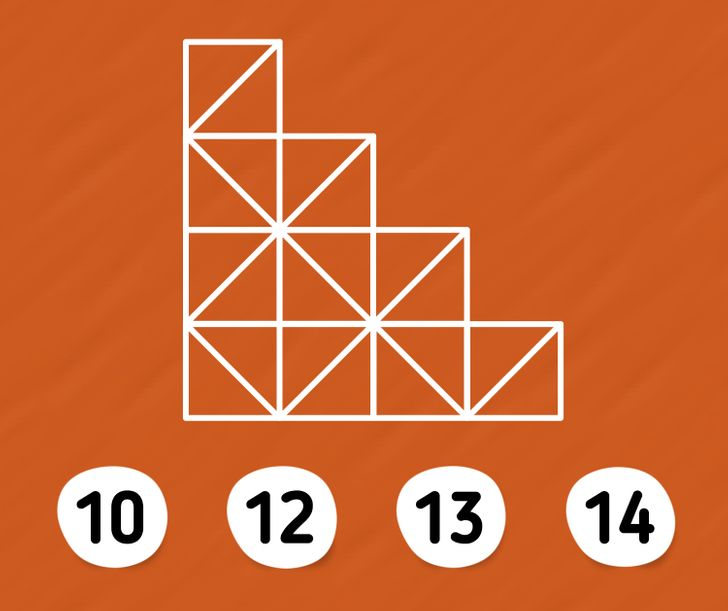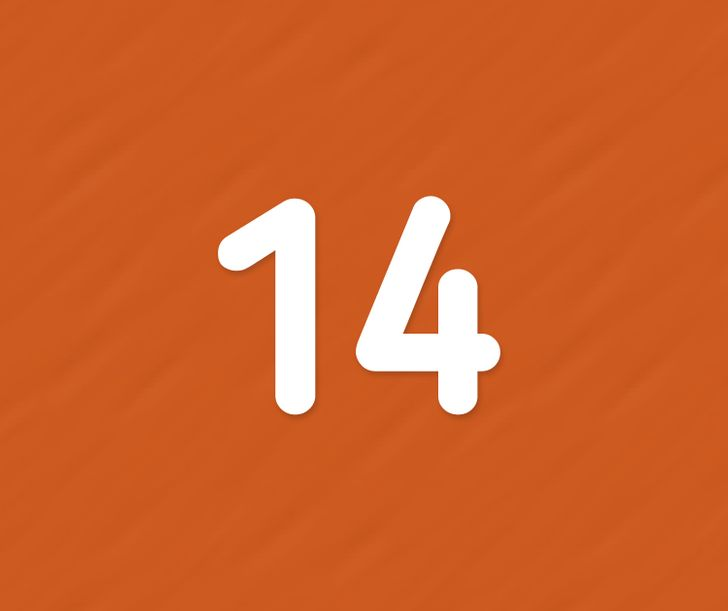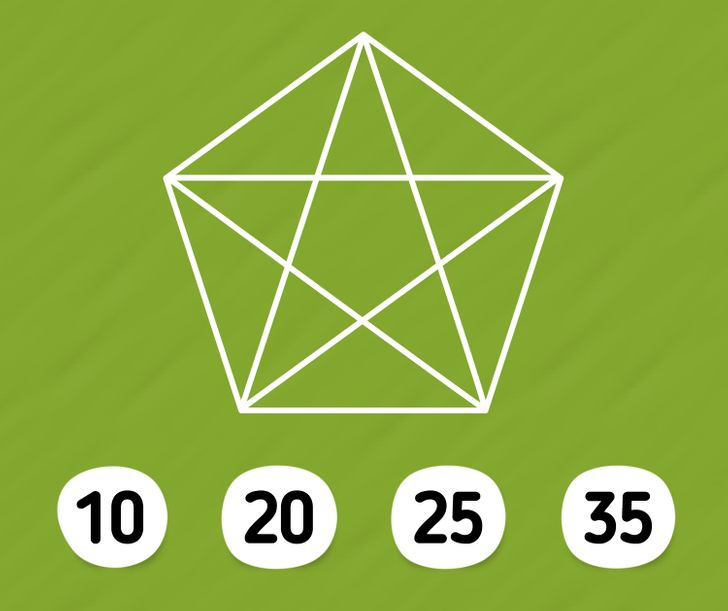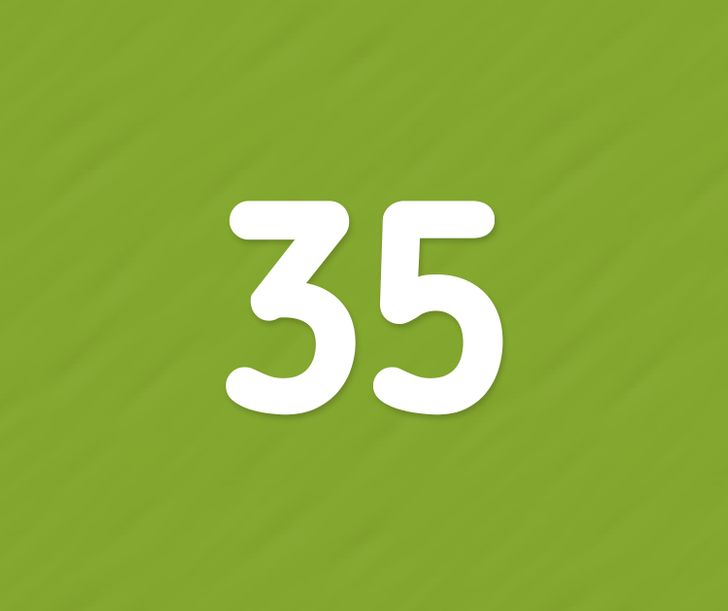# A Cunningly Simple Test to Check Your Attentiveness in Under 5 Minutes

If you’ve always wanted to give your attention skills a run for their money, then this quiz is the right one for you. This 5-minute test is stunningly simple, but it’ll still provide a general indication of how attentive you are to detail and shine a light on your natural intelligence.

We at Bright Side prepped a drill for your eyes, so buckle up, and make sure to bring your A-game. Good luck.

### 1. How many squares are in the figure?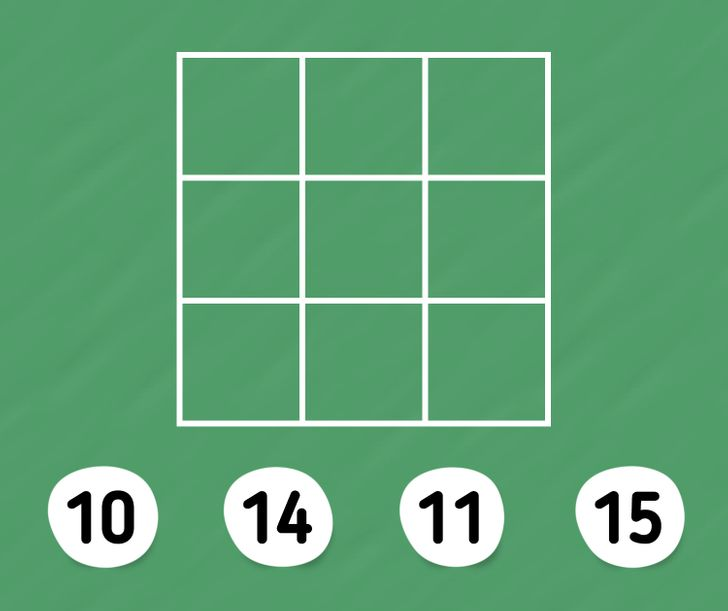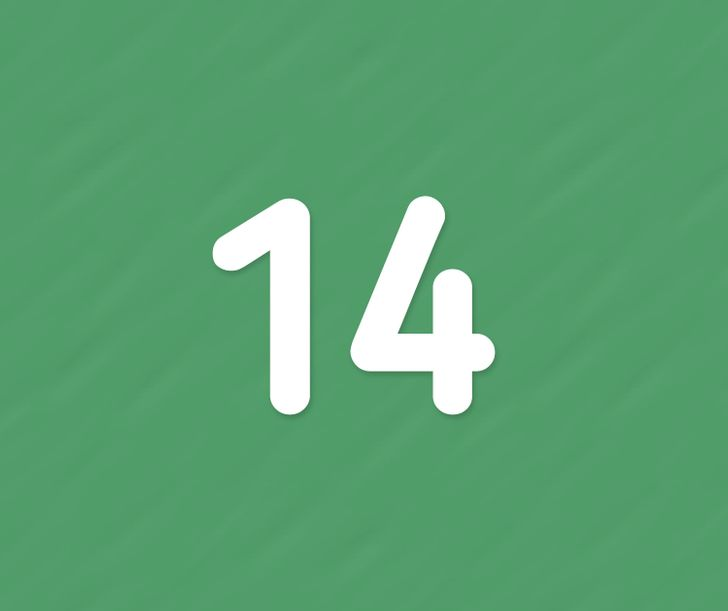### 2. And how many squares are in this one?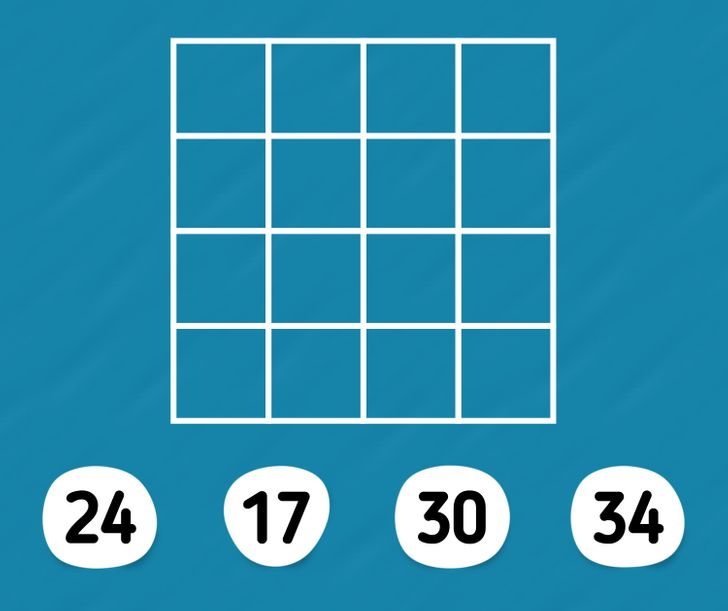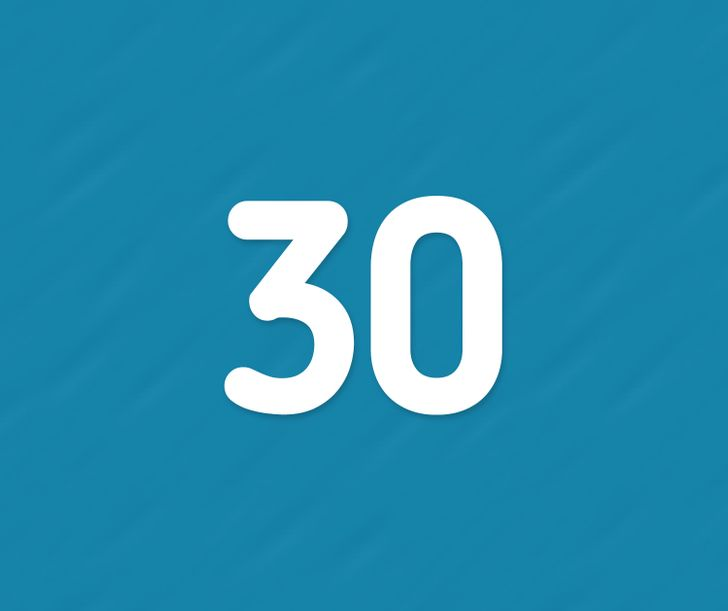### 3. How many triangles are in the figure?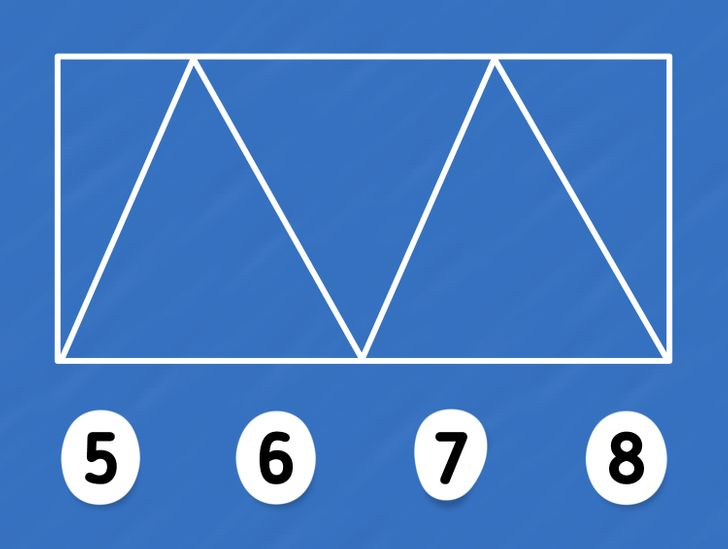### 4. And what is the number of triangles here?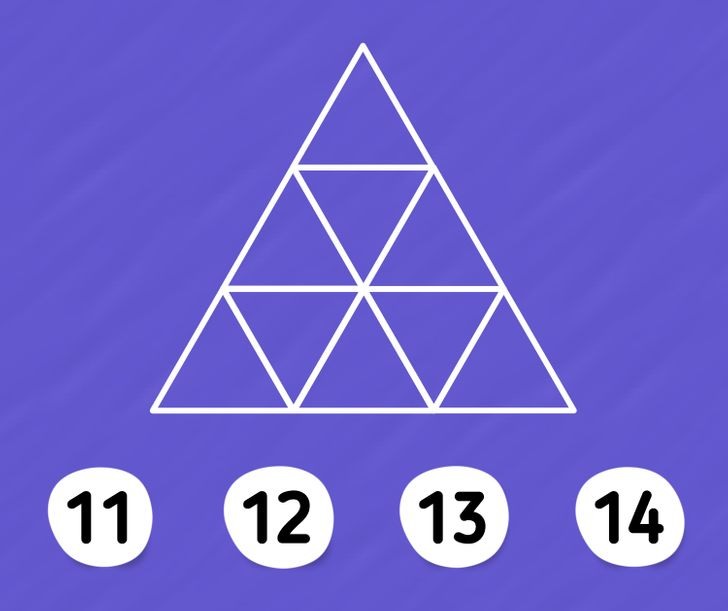### 5. And how many triangles are here?### 6. How many triangles are here?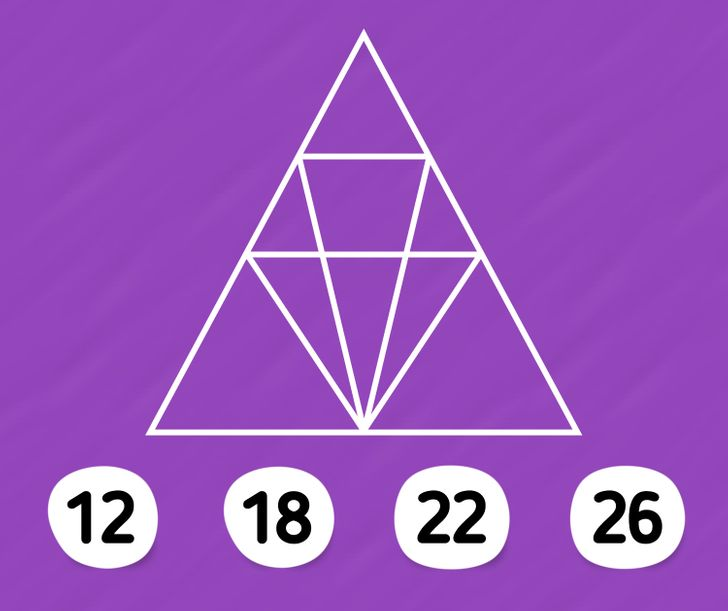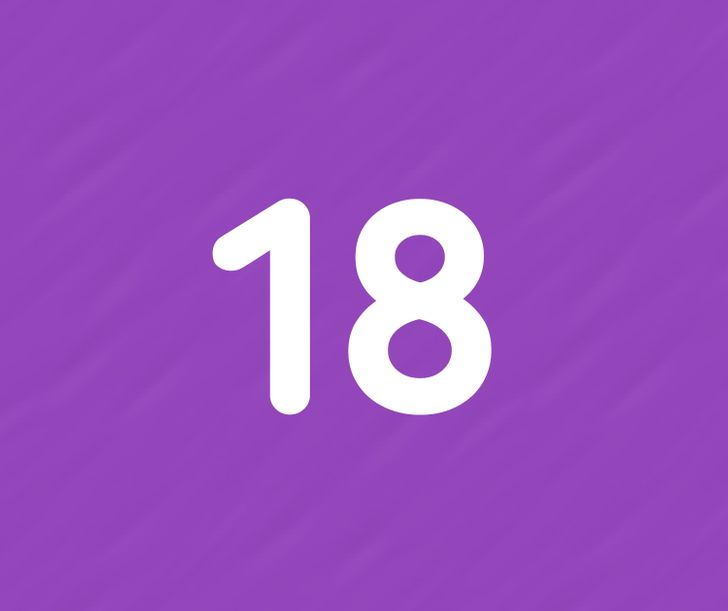### 7. How many circles are there?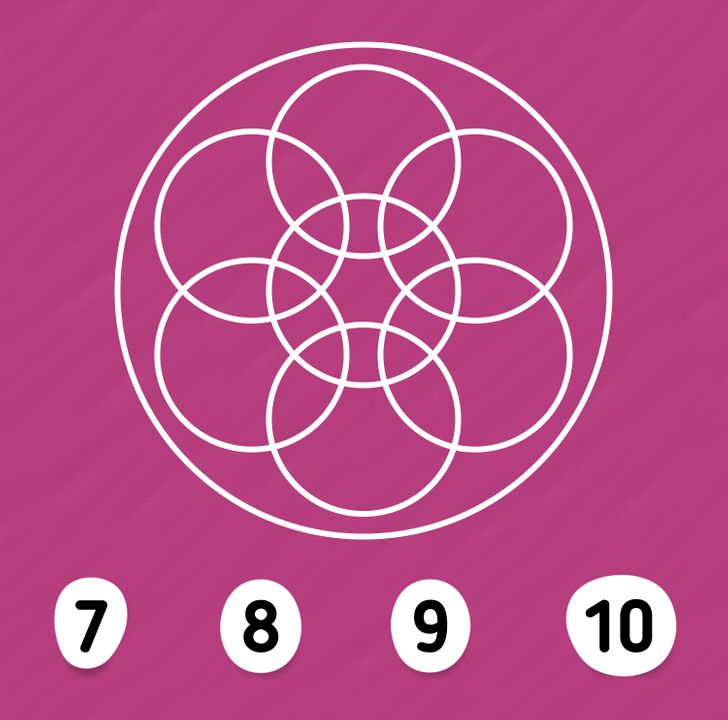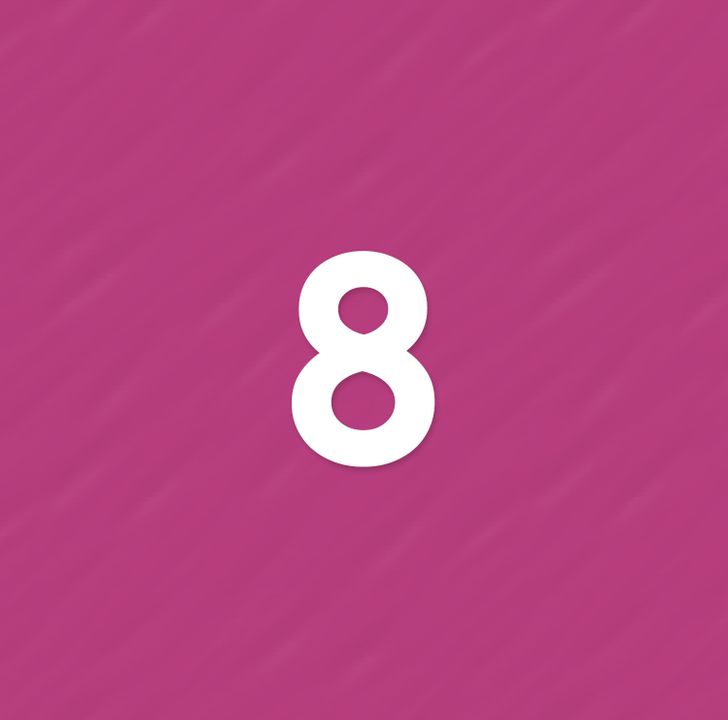### 8. How many squares are there?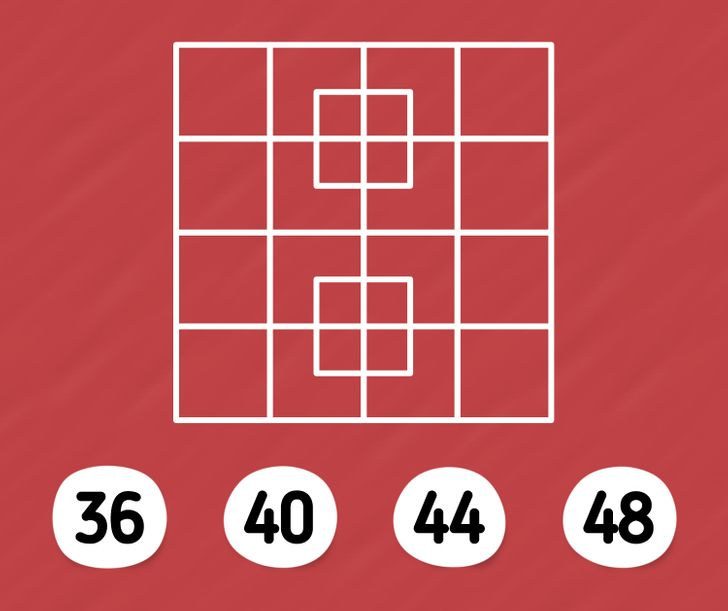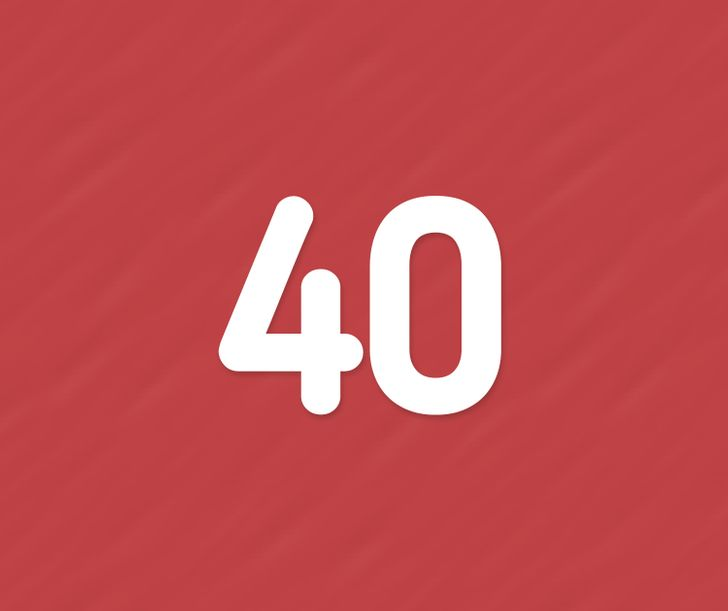### 9. How many squares are in the given figures?